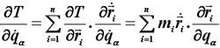Language :
 Picture : Type :[|jpg|gif|jpeg|png|] Byte :[＜2000KB] Language : 中文(简体) 中文(繁體) English 日本 한국어 Deutsch Française Ελληνικά Россию svenska Nederlandse Polska Український dansk български Italiano Icelandic român suomen kieli Galicia Türk Pilipino Català český hrvatski Latvijas Lietuvos македонски norsk språk Српски језик slovenský jazyk slovenščina Magyar nyelv فارسی Português ไทย Español Bahasa Indonesia Ngôn ngữ Việt Nam العربية Gaeilge shqiptar eesti Беларускія Die Boole-taal (Afrikaans) Malti Melayu lugha ya Kiswahili Cymraeg עברית שפה ייִדיש हिन्दी esperanto bosanski اردو زبان Azərbaycan ქართული Kreyòl ayisyen Euskal հայերեն ગુજરાતી ಕನ್ನಡ latin ພາສາລາວ বাংলা ভাষা తెలుగు தமிழ் மொழி ខ្មែរ | Check code :[Visitor (112.21.*.*)]answers [Chinese ] Time :2022-01-25Lagrange equations: Dynamic equations expressed in generalized coordinates for complete systems, usually referring to the second type of Lagrange equations, were first derived by the French mathematician J.-L. Lagrange.where T is the kinetic energy of the system expressed by each generalized coordinate qj and each generalized velocity q'j; Qj is the generalized force corresponding to qj; N (=3n-k) is the degree of freedom of this complete system; n is the number of mass points of the system; k is the number of complete constraint equations.From the virtual displacement principle, it is possible to obtain a non-binding equilibrium equation for the particle system that is ideally constrained, while the dynamic and static method (D'Alembert's principle) applies the static method of writing the equilibrium equation to establish the dynamic equation of the particle system, and the combination of the two can obtain a non-binding particle system dynamic equation, which is the general equation of dynamics. The Lagrange equation, on the other hand, is a concrete representation of the general equations of dynamics at generalized coordinates.The Lagrange equation can be used to establish non-binding kinetic equations, or it can be used to solve for the active forces acting on a system given the laws of motion of the system. For binding force , the Lagrange equations can be combined with the motion law or the momentum theorem ( or the centroid motion theorem ) .Usually, we call Newtonian's laws and the theories of mechanics based on them Newtonian mechanics (also known as vector mechanics), and the Lagrange equations and the theories on which they are built are called Lagrangian mechanics. Lagrange mechanics describes the motion of a mechanical system through a geometric space, and it is suitable for studying the motion of a constrained particle system. Lagrange mechanics plays an important role in solving the problem of micro-amplitude vibration and some problems of rigid body dynamics.